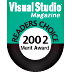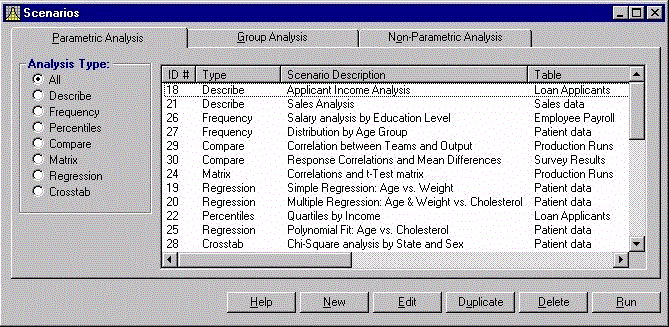Supports Microsoft Visual Basic 6.0 (VB6)

Statistics Info:

Reviewers Guide

FAQsBest Data Analysis Tool
Rave ReviewsWhy Statistics?

"The application is great! It is relatively easy to use and is great for developing programs in VB6 that require statistical calculations."

Dan Biscoe

Statistical Analysis for Visual Basic 6 (VB6) Applications

Total VB Statistics offers a wide range of statistical functions for Visual Basic 6.0 programmers using Microsoft Jet Engine databases. Any Microsoft Access/Jet table or linked table such as SQL Server, dBase, or Paradox table can be analyzed. Multi-table Select Queries can also be analyzed and an unlimited number of records can be processed in one pass.

Developed specifically for VB6 programmers, Total VB Statistics eliminates the need to export your data to other programs for statistical analysis. You can perform a wide range of statistical functions with all results in Microsoft Access tables. View, sort, query, or display the results. You can also add it to forms and reports just like any other table.

Total VB Statistics includes two parts. The first is an interactive Statistics Scenario Designer. This standalone program lets you easily specify the database, data, fields, and analysis to perform. The second part is a royalty free ActiveX DLL component that performs the analysis. This file, under 1 Meg, is the only file you need to include with your VB6 applications to add statistical analysis.The Total VB Statistics Scenario Designer

Features

• Statistically analyze data from any Jet Database or linked table
• Analyze multi-table Select queries
• Analyze an unlimited number of records
• All output in Jet tables
• No programming required to specify the analysis to perform
• Analysis settings are saved as "scenarios" which can be run again in the future
• Analyze multiple fields at one time
• Use data grouping fields to analyze groups of records (optional)
• Weight data based on a weighting field's value (optional)
• Ignore a numeric value or range of values (optional)
• Includes a royalty-free runtime license
• Scenarios are compatible with Total Access Statistics. Scenarios created in either program can be run by the other.

Calculations Available in Total VB Statistics

Field Analysis

• Count, Missing (blanks), Mean.
• Minimum, Maximum, Range, Sum, Sum of Squares.
• Variance, Coefficient of Variance, Std. Deviation, Std. Error.
• Geometric Mean, Harmonic Mean, Root Mean Square.
• Skewness, Kurtosis.
• Mode, Mode Count.
• t-Test versus Mean (test for null hypothesis).
• Confidence Intervals (t and Normal tests). 95% and user-specified levels.

Percentiles and Frequency Distributions

• Percentiles: median, quartiles, deciles, and percentiles.
• Frequency distribution with user-specified group width, initial value and number of intervals. Display count, percent of total, sums and cumulative values.

Field Comparison - Compare fields in a record

• Mean difference
• Standard Deviation of difference
• Covariance
• Correlation
• R-Square
• Paired t-Test
• Probability (1 or 2 tails).

Regression

• Simple, Multiple and Polynomial (up to 9th order).
• With Y-Intercept or Zero-Intercept.
• Count, Missing, R-Square, Std. Error of Est., and ANOVA
• Coefficient analysis: P Std. Error, Beta, t-Value and Probability.
• Residual calculation for each data point.

Cross-Tabulation and Chi-Square

• 10 types: Count, Sum, Average, Minimum, Maximum, Range, Variance, Coefficient of Variance, Std. Deviation, Std. Error.
• Count crosstabs for Text and Date value fields.
• Row total and Column sub-totals.
• Percent of Column, Row and Total.
• Sorted column fields with special handling for blank values.
• Chi-Square Analysis: Chi-Square, Probability, Cramer's V, Phi-Coefficient, Coefficient of Contingency.

Group Analysis - Compare groups of records

• Two-Sample t-Tests (pooled and separate variance tests).
• ANOVA (Analysis of Variance) with sum of squares, F-Value and probability.

Non-Parametric Analysis

• One sample Chi-Square
• One Sample Sign Test for Median and Mean
• Kolmogorov-Smirnov Goodness of Fit Test
• Paired Sign Test, Spearman's Rho Correlation
• Wilcoxon Signed Rank Test for Matched Pairs
• Wald-Wolfowitz Runs Test
• Mann-Whitney U Two Sample Test
• Kolmogorov-Smirnov Two Sample Test
• Kruskal-Wallis One Way Analysis of Variance
• Friedman's Two Way Analysis of Variance

One Sample Sign Test for Median.

• Two Sample Sign Test for Equal Median.
• Wald-Wolfowitz Sign Test for Equal Median.
• Wilcoxon Test for Matched Pairs.
• Mann-Whitney Two Sample (non-matched) Test.
• Kolmogorov-Smirnov Two Sample Test.
• Correlations: Spearman's Rho and Kendall's Tau.
• Kruskal-Wallis One Way Analysis of Variance.
• Friedman's Two Way Analysis of Variance.

Probability Calculator

• Evaluate probability of test values: Z, t, Chi-Square, and F.
Enter the test value and degrees of freedom to see the corresponding probability.
• Calculate inverse probability. Enter a probability and calculate the equivalent Z, t, Chi-Square, and F value.
• Eliminate interpolating values in reference tables.
 Total VB Statistics License Price Visual Basic 6.0Single \$5995-Seat \$1799Premium Support SubscriptionSingle \$299 More Information5 Seat \$999 Also Available as part of: Total VB Enterprise Suite Runtime/Redistributable Version Royalty-free runtime rights are included in the purchase price. Licensing Information Total VB Statistics is licensed on a per developer basis. Each developer who uses or redistributes the program must have a license.